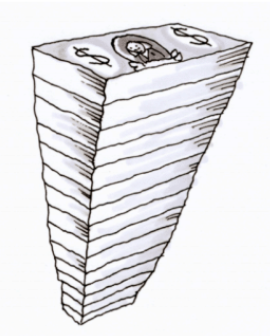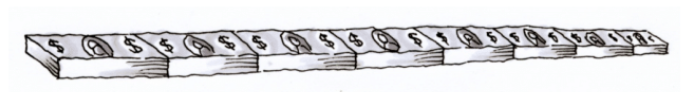### Home > MC1 > Chapter 1 > Lesson 1.1.3 > Problem1-26

1-26.

According to the U.S. Treasury, a stack of $233$ one-dollar bills is one inch high. One bill is about three inches wide and about six inches long. Will one million dollars fit in your classroom? Answer the following questions to find out.1. If your classroom is $10$ feet high, how many dollar bills in one stack will it take to reach the ceiling? Show your work.Each of these parts represents one foot, which is equal to $12$ inches.
How many bills should go in each part?

Each foot has $12$ inches, each inch has $233$ bills.

This is one inch, which is equal to $233$ bills. How many of these go into one foot? $10$ feet?

$\left(233 \text{ bills}\right)\left(12 \text{ inches}\right)\left(10 \text{ feet}\right) = 27,960 \text{ bills}$1. If the length of your classroom is $30$ feet, how many dollar bills (arranged in lengthwise stacks along the floor of one wall) will it take to cover an entire wall?

Two dollar bills fit lengthwise into one foot. We discovered that each stack has $27,960$ one-dollar bills.

This one is very similar to the last one. Try drawing a diagram if that helps you. The answer is $1,677,600$.

2. If the dimensions of your classroom are $30$ feet by $20$ feet by $10$ feet, how many dollar bills will it take to fill the room? Show all of your work.

Four stacks of bills can fit in a foot if you line up their widths ($3$ inches).